Next: Building circuits with op-amps Up: Operational Amplifiers: Basic Concepts Previous: Voltage follower

# Follower with gain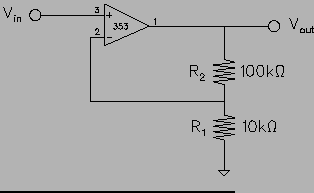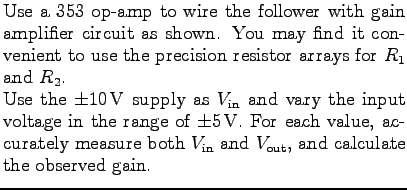Plot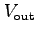vs.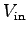, determine the region of linearity, and fit that part of the data to a straight line to determine gain. Note that you must allow for a non-zero intercept, thus use a two-parameter fit. Compare the gain you obtain with the values calculated at each data point, and with the value you would expect for this circuit from the nominal resistor values.

Explain what limits the gain for large values of.

Use the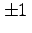V supply as input, set at slightly below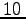mV. Vary the gain of the circuit by using different values of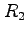: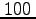k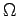,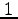M, andM.

Compare the measured gains to those expected and explain any deviations.

Can a gain of less than 1 be obtained with this circuit? Explain why or why not.

In the above plot, the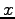-intercept of the straight line corresponds to the input offset voltage,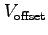. It may be more clearly observed at low input voltages and high gains. Examine this effect by connecting the follower input to common and measuring, for the samevalues as above. Be sure to disconnect the voltage source from the follower input before grounding the latter!GainkMMCompare yourvalue to the-intercept above and comment on differences, if any.

What causes a greater % deviation from nominal gain,or the resistor inaccuracy?

Optional: Repeat the straight-line fit to the data, but this time force the intercept to be zero (i.e., a one-parameter fit). This assumes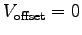. Calculate and comment on % error caused by this assumption.Next: Building circuits with op-amps Up: Operational Amplifiers: Basic Concepts Previous: Voltage follower

For info, write to: physics@brocku.ca
Last revised: 2007-01-05